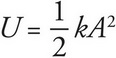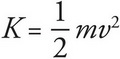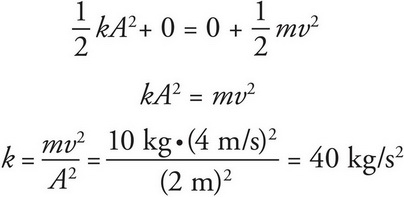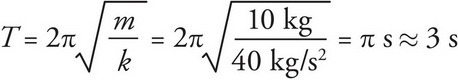# AP Physics 1 Question 253: Answer and Explanation

### Test Information

Question: 253

8. A block with a mass of 10 kg connected to a spring oscillates back and forth with an amplitude of 2 m. What is the approximate period of the block if it has a speed of 4 m/s when it passes through its equilibrium point?

• A. 1 s
• B. 3 s
• C. 6 s
• D. 12 s

By Conservation of Mechanical Energy, the energy of the block is the same throughout the motion. At the amplitude, the block has potential energyand zero kinetic energy. At the equilibrium position, the block has kinetic energyand zero potential energy. Applying the Conservation of Mechanical Energy to these two points in the motion yields